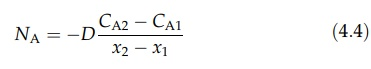# Molecular Diffusion in Liquids

| Home | | Pharmaceutical Technology |

## Chapter: Pharmaceutical Engineering: Mass Transfer

Equations describing molecular diffusion in liquids are similar to those applied to gases.

MOLECULAR DIFFUSION IN LIQUIDS

Equations describing molecular diffusion in liquids are similar to those applied to gases. The rate of diffusion of material A in a liquid is given by equation (4.1).Fick’s law for steady-state, equimolal counterdiffusion is thenwhere CA2 and CA1 are the molar concentrations at points x2 and x1, respectively. Equations for diffusion through a layer of stagnant liquid can also be developed. The use of these equations is, however, limited because diffusivity in a liquid varies with concentration. In addition, unless the solutions are very dilute, the total molar concentration will vary from point to point. These complications do not arise with diffusion in gases.

Diffusivities in liquids are very much less than diffusivities in gases, commonly by a factor of 104.